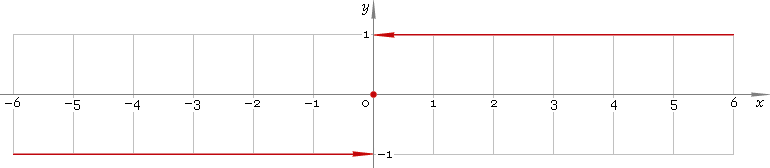The Art of Mathematics

# sign — signum function

## 1. Definition

Signum function is defined as

sign(x) ≡ −1, for x < 0;
sign(0) ≡ 0;
sign(x) ≡ 1, for x > 0.

## 2. Graph

Signum function is defined everywhere on real axis — so its domain is (−∞, +∞). Function graph is depicted below — fig. 1.Fig. 1. Graph of the signum function y = signx.

Function codomain is limited to the set of values {−1, 0, 1}.

## 3. How to use

To get signum of the number:

``sign(−1.7);``

To get signum of the complex number:

``sign(−1.7+i);``

To get signum of the current result:

``sign(rslt);``

To get signum of the number z in calculator memory:

``sign(mem[z]);``

## 5. Support

Signum of the real argument is supported in free version of the Librow calculator.

Signum of the complex argument is supported in professional version of the Librow calculator.